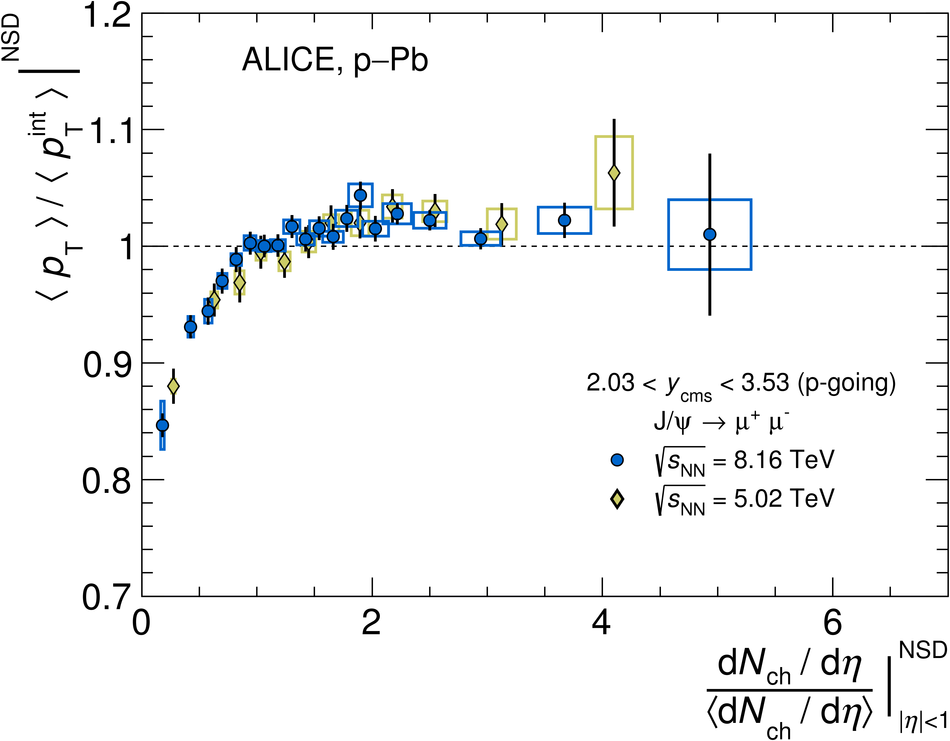# J/$ψ$ production as a function of charged-particle multiplicity in p-Pb collisions at $\sqrt{\textit{s}_{\rm NN}}~=~8.16$ TeV

Inclusive J/$\psi$ yields and average transverse momenta in p-Pb collisions at a center-of-mass energy per nucleon pair $\sqrt{s_{\rm NN}}$ = 8.16 TeV are measured as a function of the charged-particle pseudorapidity density with ALICE. The J/$\psi$ mesons are reconstructed at forward $(2.03 <~ y_{\rm cms} <~ 3.53)$ and backward ($-4.46 <~ y_{\rm cms} <~ -2.96$) center-of-mass rapidity in their dimuon decay channel while the charged-particle pseudorapidity density is measured around midrapidity. The J/$\psi$ yields at forward and backward rapidity normalized to their respective average values increase with the normalized charged-particle pseudorapidity density, the former showing a weaker increase than the latter. The normalized average transverse momenta at forward and backward rapidity manifest a steady increase from low to high charged-particle pseudorapidity density with a saturation beyond the average value.

Submitted to: JHEP
e-Print: arXiv:2004.12673 | PDF | inSPIRE

Figures

## Figure 1

 Opposite-sign muon pair invariant mass distributions for selected multiplicity intervals, corrected for the $\Jpsi$ acceptance and efficiency, at forward rapidity. The distributions are shown together with a typical fit function (solid line, see text for details). The $\Jpsi$ signal contribution is also depicted by a dot-dashed red line, and the background by a dotted line.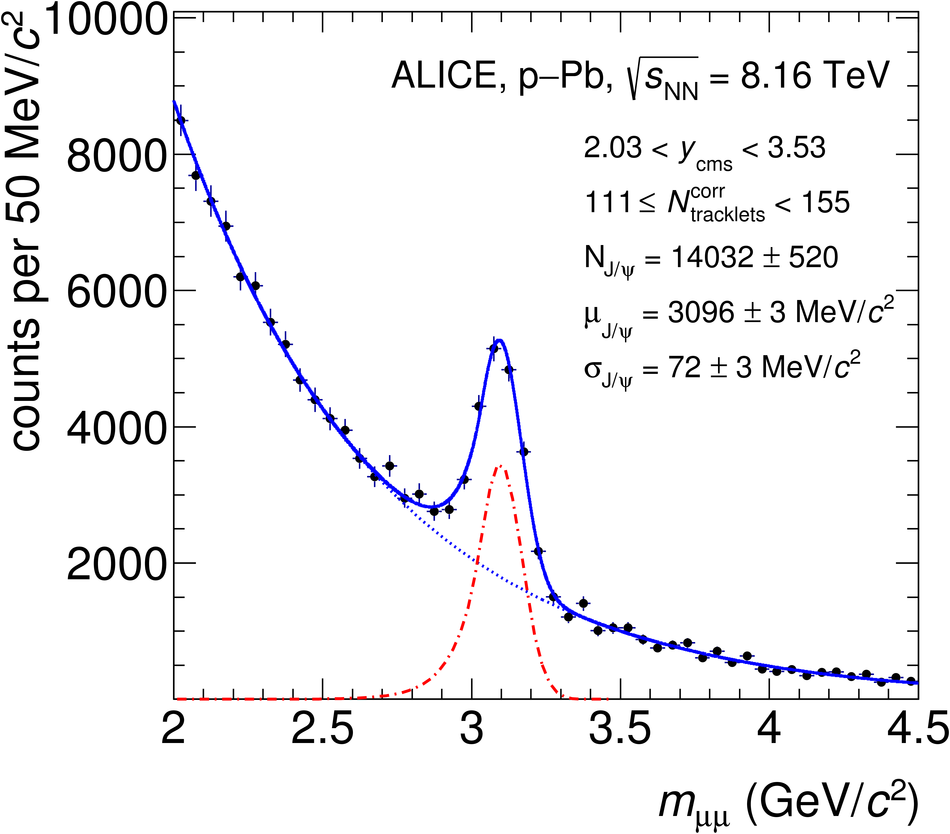## Figure 2

 Average transverse momentum of opposite-sign muon pairs for selected multiplicity intervals, corrected for the $\Jpsi$ acceptance and efficiency. The distributions are shown together with a typical fit function (see text for details)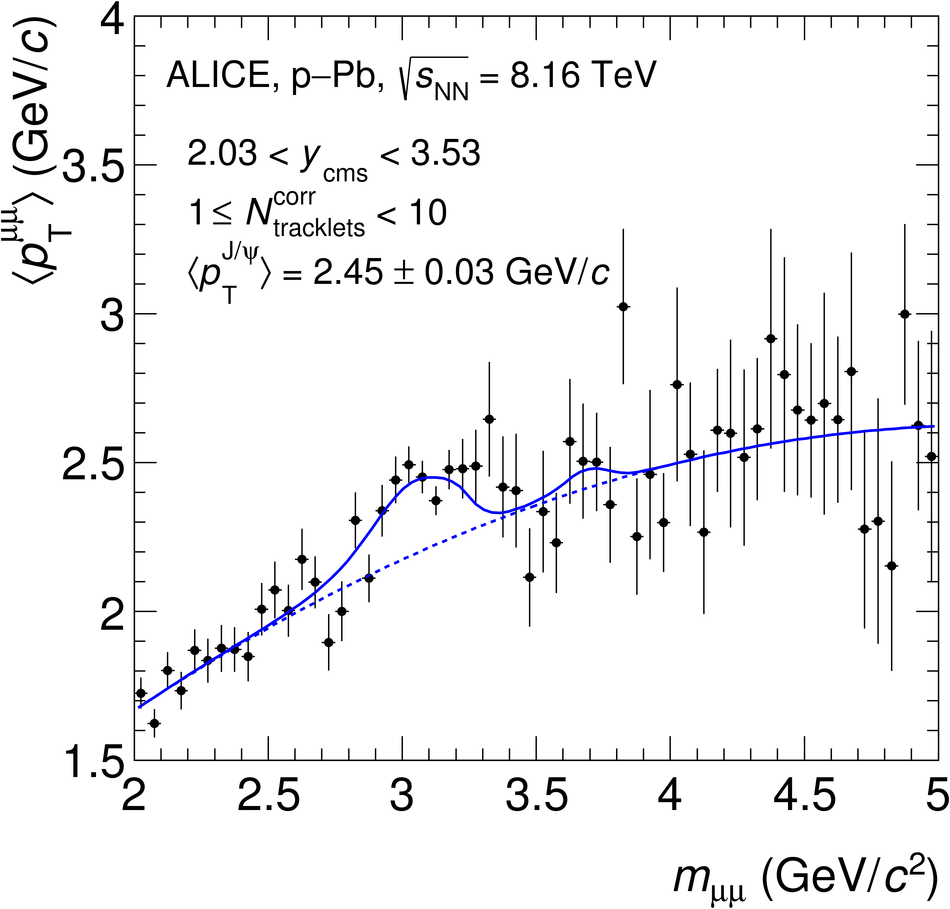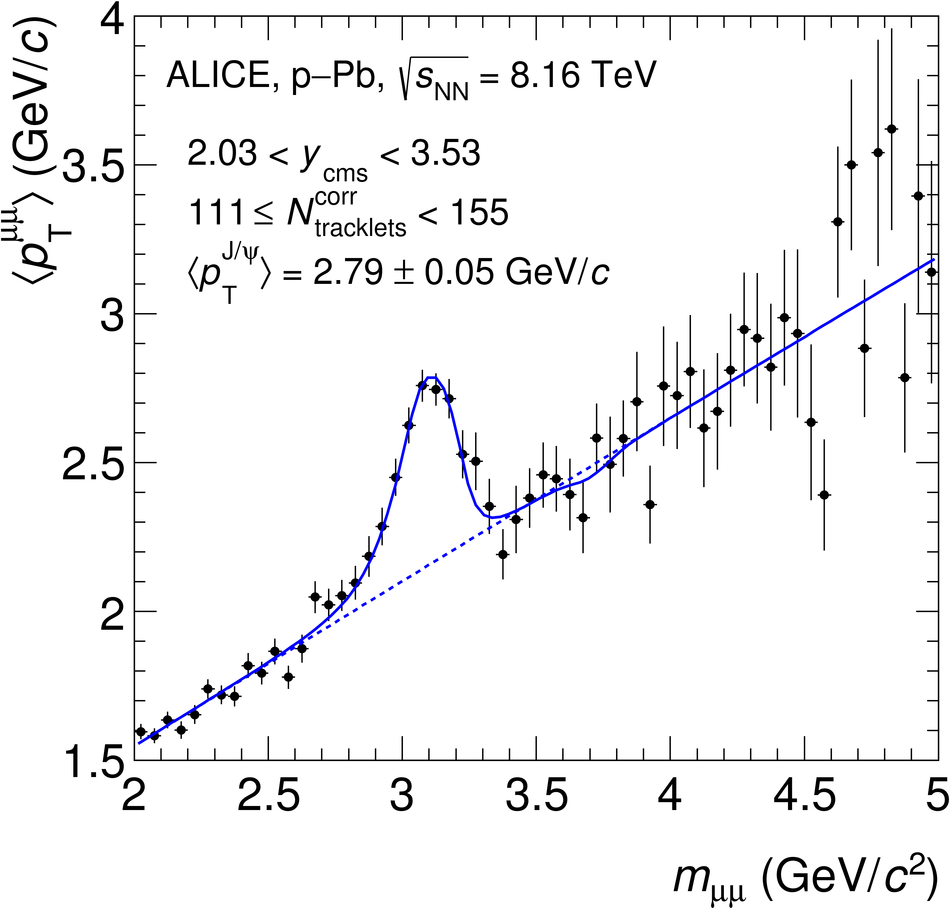## Figure 3

 Normalized yield of inclusive $\Jpsi$, at forward and backward rapidities, as a function of the normalized charged-particle pseudorapidity density, measured at midrapidity, in \pPb collisions at \eightnnNS. The vertical bars represent the statistical uncertainties. The vertical and horizontal widths of the boxes represent the respective systematic uncertainties for the $\Jpsi$ yields and the multiplicities The dashed line indicates the diagonal line, to guide the eye## Figure 4

 Normalized yield of inclusive $\Jpsi$ as a function of the normalized charged-particle pseudorapidity density, measured at midrapidity, in \pPb collisions at \eightnnNS~and \fivenn~. The top (bottom) panel presents the measurement at forward (backward) rapidity The vertical bars represent the statistical uncertainties, the boxes the systematic ones. The dashed line indicates the diagonal line, to guide the eye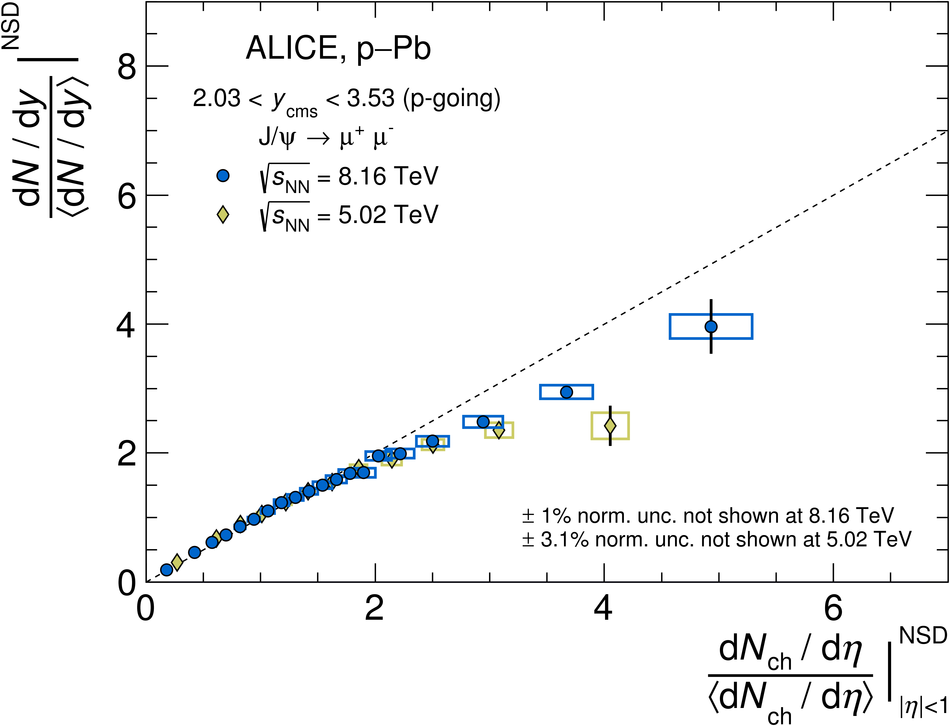## Figure 5

 Top: Normalized yield of inclusive $\Jpsi$ as a function of the normalized charged-particle pseudorapidity density, measured at midrapidity, in various collision systems. Bottom: Ratio of the normalized yields to the corresponding normalized charged-particle pseudorapidity density. The pp results are normalized to INEL collisions~, whereas \pPb~ones refer to the NSD event class; all for $\pt>0$. The Pb--Pb data points include $\Jpsi$ with $0.3< \pt< 12~{\rm GeV}/c$ to reduce the low-$\pt$ contribution from photoproduction, which is significant only in more peripheral collisions~ The vertical bars represent the statistical uncertainties, the boxes the systematic ones. The dashed line indicates the diagonal line, to guide the eye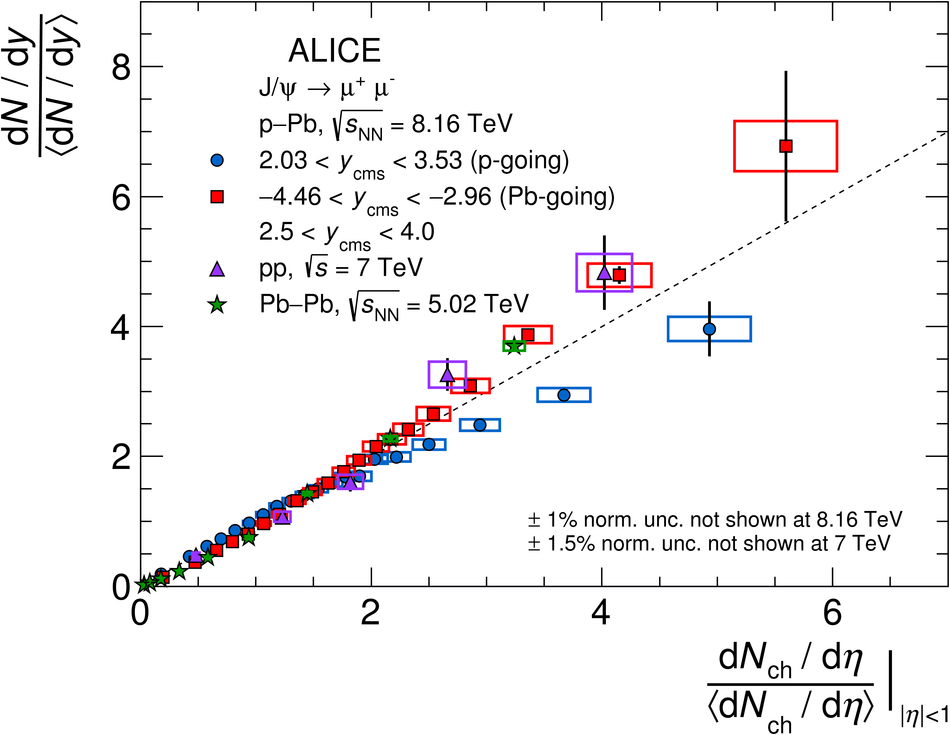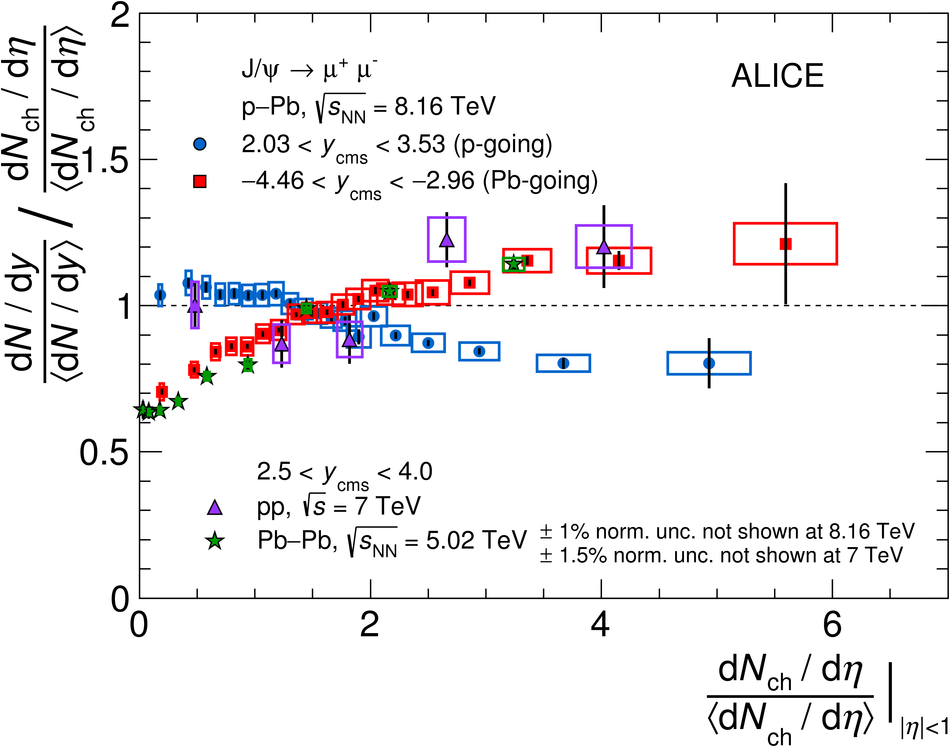## Figure 6

 Normalized yield of inclusive $\Jpsi$ as a function of the normalized charged-particle pseudorapidity density, measured at midrapidity, in \pPb collisions at \eightnnNS~compared with EPOS~3~ calculations. Left (right) panel presents the measurement at forward (backward) rapidity The vertical bars represent the statistical uncertainties, the boxes the systematic ones. The dashed line indicates the diagonal line, to guide the eye. The shaded areas represent the statistical uncertainties on the EPOS~3 calculations.## Figure 7

 Average transverse momentum of inclusive $\Jpsi$ at forward and backward rapidities as a function of the normalized charged-particle pseudorapidity density, measured at midrapidity, in \pPb collisions at \eightnnNS. The vertical bars represent the statistical uncertainties, the boxes the systematic ones.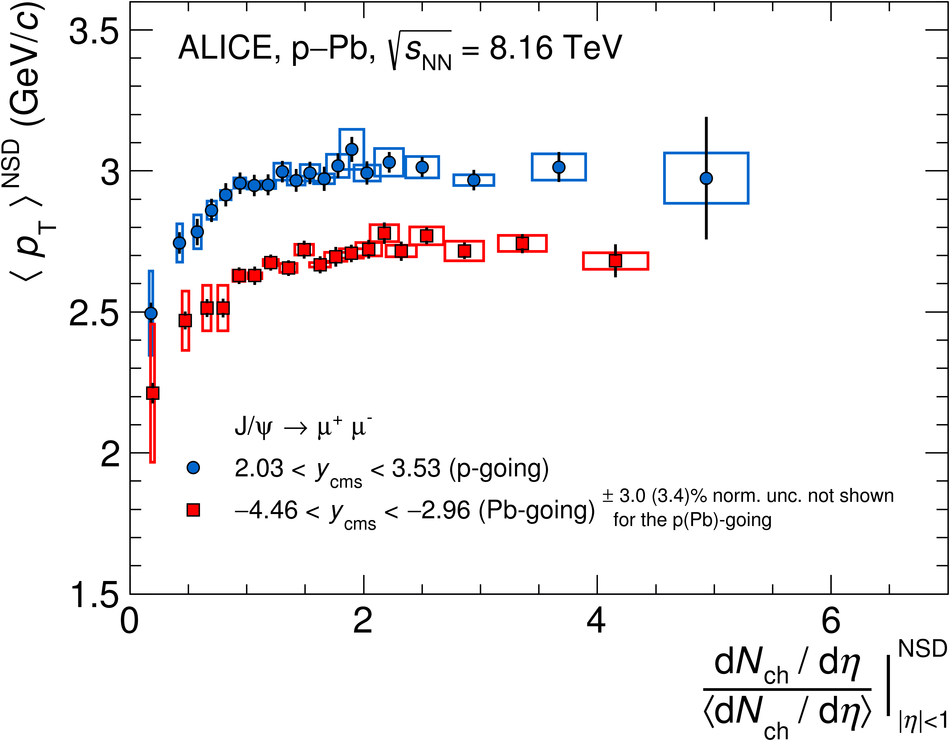## Figure 8

 Normalized average transverse momentum of inclusive $\Jpsi$ as a function of the normalized charged-particle pseudorapidity density, measured at midrapidity, in \pPb collisions at \eightnnNS~and \fivenn~. Top (bottom) panel presents the measurement at forward (backward) rapidity The vertical bars represent the statistical uncertainties, the boxes the systematic ones.